JMSLTM Numerical Library 7.2.0
com.imsl.stat

## Class ARSeasonalFit

• All Implemented Interfaces:
Serializable

```public class ARSeasonalFit
extends Object
implements Serializable```
Estimates the optimum seasonality parameters for a time series using an autoregressive model, AR(p), to represent the time series.

ARMA time series modeling assumes the time series is stationary. Seasonal trends and cycles violate this assumption, which can lead to inaccurate predictions. However, in many cases the nonstationary series can be transformed into a stationary series by first differencing the series. For example, if the correlation is strong from one period to the next, the series might be differenced by a lag of 1. Instead of fitting a model to the original series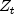, the model is fitted to the transformed series: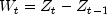. Higher order lags or differences are warranted if the series has cycles every 4 or 13 weeks. Class `ARSeasonalFit` is designed to help identify the optimum differencing for a series with seasonal trends or cycles.

`ARSeasonalFit` assumes the original series has no missing values, is equally spaced in time and is not centered before computing the optimum differencing. However, by default the transformed series is centered using the mean of that series. Users can change this default using the `setCenter` method. If `setCenter` is set to ``` NO_CENTER``` the series is not centered, if set to `CENTER_MEAN` the series is centered using the mean of the series, and if set to `CENTER_MEDIAN`, the series is centered using the median of the series. If `setCenter` is set to `CENTER_MEAN` or `CENTER_MEDIAN` then the differenced series,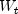is centered before determination of minimum AIC and optimum lag.

For every combination of rows in `sInitial` and ```dInitial ```, the seriesis converted to the seasonally adjusted series using the following computation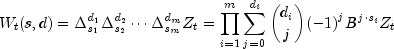where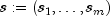,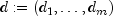represent specific rows of arrays ```sInitial ``` and `dInitial` respectively, and m is the number of differences, or m`=sInitial.length`.

This transformation of the seriesto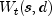is computed using the `Difference` class. After this transformation the transformed seriesis centered, unless `NO_CENTER` is specified, and the `ARAutoUnivariate` class is used to automatically determine the optimum lag for an AR(p) representation for.

This procedure is repeated for every possible combination of rows in `sInitial` and `dInitial`. The series with the minimum AIC is identified as the optimum representation and returned in the methods `getAROrder`, `getOptimumS`, `getOptimumD`, `getAIC`, and `getAR`. The transformed series with the minium AIC can be retrieved from the ``` getTransformedTimeSeries``` method.

Example, Serialized Form
• ### Field Summary

Fields
Modifier and Type Field and Description
`static int` `CENTER_MEAN`
Indicates the transformed series should be centered using the average of the differenced series.
`static int` `CENTER_MEDIAN`
Indicates the transformed series should be centered using the median of the differenced series.
`static int` `NO_CENTER`
Indicates the transformed series should not be centered.
• ### Constructor Summary

Constructors
Constructor and Description
```ARSeasonalFit(int maxlag, int[][] sInitial, double[] z)```
Constructor for `ARSeasonalFit`.
• ### Method Summary

Methods
Modifier and Type Method and Description
`void` `compute()`
Computes the minimum AIC and optimum values for s and d based upon the candidates provided in `sInitial` and ``` dInitial```, and computes the values for the transformed series,.
`double` `getAIC()`
Returns the final estimate for Akaike's Information Criterion (AIC) at the optimum.
`double[]` `getAR()`
Returns the final autoregressive parameter estimates at the optimum in the transformed series.
`int` `getAROrder()`
Returns optimum number of lags, p, for the optimum autoregressive AR(p) model.
`int` `getCenter()`
Returns the current setting for centering the input time series.
`int[][]` `getDInitial()`
Returns the candidate values for d to evaluate.
`boolean` `getExclude()`
Returns the current setting for excluding or replacing the inital values in the transformed series.
`int` `getMaxlag()`
Returns the maximum lag used to fit the AR(p) model.
`int` `getNLost()`
Returns the number of values in the initial part of the series lost to differencing.
`int[]` `getOptimumD()`
Returns the optimum values for d selected among the candidates in `dInitial`.
`int[]` `getOptimumS()`
Returns the optimum values for s selected among the candidates in `sInitial`.
`int[][]` `getSInitial()`
Returns the the candidate values for s to evaluate.
`double[]` `getTimeSeries()`
Returns the time series.
`double[]` `getTransformedTimeSeries()`
Returns the transformed series,.
`void` `setCenter(int center)`
Controls centering of the differenced series.
`void` `setDInitial(int[][] dInitial)`
Sets the candidate values for selecting the optimum seasonal adjustment prior to calling the compute method.
`void` `setExclude(boolean exclude)`
Controls whether to exclude or replace the inital values in the transformed series.
• ### Methods inherited from class java.lang.Object

`clone, equals, finalize, getClass, hashCode, notify, notifyAll, toString, wait, wait, wait`
• ### Field Detail

• #### CENTER_MEAN

`public static final int CENTER_MEAN`
Indicates the transformed series should be centered using the average of the differenced series.
Constant Field Values
• #### CENTER_MEDIAN

`public static final int CENTER_MEDIAN`
Indicates the transformed series should be centered using the median of the differenced series.
Constant Field Values
• #### NO_CENTER

`public static final int NO_CENTER`
Indicates the transformed series should not be centered.
Constant Field Values
• ### Constructor Detail

• #### ARSeasonalFit

```public ARSeasonalFit(int maxlag,
int[][] sInitial,
double[] z)```
Constructor for `ARSeasonalFit`.
Parameters:
`maxlag` - an `int` scalar specifying the maximum lag allowed when fitting an AR(p) model.
`sInitial` - an `int` matrix where each row represents seasonal differences to evaluate. The number of columns in `sInitial` represent the number of differences to perform. All values of ```sInitial ``` must be greater than zero.
`z` - an input `double` array containing the time series.
• ### Method Detail

• #### compute

```public void compute()
throws ARMA.MatrixSingularException,
ARMA.TooManyCallsException,
ARMA.IncreaseErrRelException,
ARMA.NewInitialGuessException,
ARMA.IllConditionedException,
ARMA.TooManyITNException,
ARMA.TooManyFcnEvalException,
ARMA.TooManyJacobianEvalException,
ARAutoUnivariate.TriangularMatrixSingularException,
ARMAMaxLikelihood.NonInvertibleException,
ARMAMaxLikelihood.NonStationaryException,
ARMAMaxLikelihood.InitialMAException```
Computes the minimum AIC and optimum values for s and d based upon the candidates provided in `sInitial` and ``` dInitial```, and computes the values for the transformed series,.

Warnings are printed if a row in `sInitial` is skipped due to too many observations lost in the differenced series or if problems occurred computing the optimum AIC using the differenced series.

Throws:
`ARMA.MatrixSingularException` - is thrown if the input matrix is singular
`ARMA.TooManyCallsException` - is thrown if the number of calls to the function has exceeded the maximum number of iterations times the number of moving average (MA) parameters + 1.
`ARMA.IncreaseErrRelException` - is thrown if the bound for the relative error is too small
`ARMA.NewInitialGuessException` - is thrown if the iteration has not made good progress
`ARMA.IllConditionedException` - is thrown if the problem is ill-conditioned
`ARMA.TooManyITNException` - is thrown if the maximum number of iterations is exceeded
`ARMA.TooManyFcnEvalException` - is thrown if the maximum number of function evaluations is exceeded
`ARMA.TooManyJacobianEvalException` - is thrown if the maximum number of Jacobian evaluations is exceeded
`ARMAMaxLikelihood.NonStationaryException` - is thrown if the final maximum likelihood estimates for the time series are nonstationary
`ARMAMaxLikelihood.NonInvertibleException` - is thrown if the final maximum likelihood estimates for the time series are noninvertible
`ARMAMaxLikelihood.InitialMAException` - is thrown if the initial values provided for the moving average terms using ``` setMA``` are noninvertible. In this case, ``` ARMAMaxLikelihood ``` terminates and does not compute the time series estimates.
`ARAutoUnivariate.TriangularMatrixSingularException` - is thrown if the input triangular matrix is singular.
• #### getAIC

`public double getAIC()`
Returns the final estimate for Akaike's Information Criterion (AIC) at the optimum.
Returns:
a `double` equal to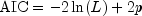, where L is the value of the maximum likelihood function evalutated at the parameter estimates.
• #### getAR

```public double[] getAR()
throws ARMA.MatrixSingularException,
ARMA.TooManyCallsException,
ARMA.IncreaseErrRelException,
ARMA.NewInitialGuessException,
ARMA.IllConditionedException,
ARMA.TooManyITNException,
ARMA.TooManyFcnEvalException,
ARMA.TooManyJacobianEvalException,
ARAutoUnivariate.TriangularMatrixSingularException,
ARMAMaxLikelihood.NonInvertibleException,
ARMAMaxLikelihood.NonStationaryException,
ARMAMaxLikelihood.InitialMAException```
Returns the final autoregressive parameter estimates at the optimum in the transformed series.
Returns:
a `double` array containing the estimates for the autoregressive parameters
Throws:
`ARMA.MatrixSingularException` - is thrown if the input matrix is singular
`ARMA.TooManyCallsException` - is thrown if the number of calls to the function has exceeded the maximum number of iterations times the number of moving average (MA) parameters + 1.
`ARMA.IncreaseErrRelException` - is thrown if the bound for the relative error is too small
`ARMA.NewInitialGuessException` - is thrown if the iteration has not made good progress
`ARMA.IllConditionedException` - is thrown if the problem is ill-conditioned
`ARMA.TooManyITNException` - is thrown if the maximum number of iterations is exceeded
`ARMA.TooManyFcnEvalException` - is thrown if the maximum number of function evaluations is exceeded
`ARMA.TooManyJacobianEvalException` - is thrown if the maximum number of Jacobian evaluations is exceeded
`ARMAMaxLikelihood.NonStationaryException` - is thrown if the final maximum likelihood estimates for the time series are nonstationary
`ARMAMaxLikelihood.NonInvertibleException` - is thrown if the final maximum likelihood estimates for the time series are noninvertible
`ARMAMaxLikelihood.InitialMAException` - is thrown if the initial values provided for the moving average terms using ``` setMA``` are noninvertible. In this case, ``` ARMAMaxLikelihood ``` terminates and does not compute the time series estimates.
`ARAutoUnivariate.TriangularMatrixSingularException` - is thrown if the input triangular matrix is singular.
• #### getAROrder

`public int getAROrder()`
Returns optimum number of lags, p, for the optimum autoregressive AR(p) model. This is the value of p for the transformed series,.
Returns:
an `int` containing the optimum number of lags in the autoregressive model used to fit the transformed series.
• #### getCenter

`public int getCenter()`
Returns the current setting for centering the input time series.
Returns:
an `int` containing the setting for center equal to 0, 1, or 2, which implies `NO_CENTER`, `CENTER_MEAN`, `CENTER_MEDIAN` respectively.
• #### getDInitial

`public int[][] getDInitial()`
Returns the candidate values for d to evaluate.
Returns:
an `int` matrix containing the candidate values for d to evaluate
• #### getExclude

`public boolean getExclude()`
Returns the current setting for excluding or replacing the inital values in the transformed series.

If exclude is `true`, then inital values in the transformed series that cannot be computed are set to missing, `NaN`. This ensures that the length of the transformed seriesis equal to the length of the time series, ```z.length ```. If exclude is set to `false`, then inital values in the transformed seriesthat cannot be computed are removed. This makes the length of the transformed seriesequal to `z.length-`nLost where nLost is the number of lost values obtained from method `getNLost`.

• #### getMaxlag

`public int getMaxlag()`
Returns the maximum lag used to fit the AR(p) model.
Returns:
an `int` scalar containing the maximum lag allowed when fitting an AR(p) model
• #### getNLost

```public int getNLost()
throws ARMA.MatrixSingularException,
ARMA.TooManyCallsException,
ARMA.IncreaseErrRelException,
ARMA.NewInitialGuessException,
ARMA.IllConditionedException,
ARMA.TooManyITNException,
ARMA.TooManyFcnEvalException,
ARMA.TooManyJacobianEvalException,
ARAutoUnivariate.TriangularMatrixSingularException,
ARMAMaxLikelihood.NonInvertibleException,
ARMAMaxLikelihood.NonStationaryException,
ARMAMaxLikelihood.InitialMAException```
Returns the number of values in the initial part of the series lost to differencing.
Returns:
an `int` containing the number of values in the initial part of the series lost to differencing. These lost values will be set to missing or dropped in the transformed series, depending upon the setting for exclude.
Throws:
`ARMA.MatrixSingularException` - is thrown if the input matrix is singular
`ARMA.TooManyCallsException` - is thrown if the number of calls to the function has exceeded the maximum number of iterations times the number of moving average (MA) parameters + 1.
`ARMA.IncreaseErrRelException` - is thrown if the bound for the relative error is too small
`ARMA.NewInitialGuessException` - is thrown if the iteration has not made good progress
`ARMA.IllConditionedException` - is thrown if the problem is ill-conditioned
`ARMA.TooManyITNException` - is thrown if the maximum number of iterations is exceeded
`ARMA.TooManyFcnEvalException` - is thrown if the maximum number of function evaluations is exceeded
`ARMA.TooManyJacobianEvalException` - is thrown if the maximum number of Jacobian evaluations is exceeded
`ARMAMaxLikelihood.NonStationaryException` - is thrown if the final maximum likelihood estimates for the time series are nonstationary
`ARMAMaxLikelihood.NonInvertibleException` - is thrown if the final maximum likelihood estimates for the time series are noninvertible
`ARMAMaxLikelihood.InitialMAException` - is thrown if the initial values provided for the moving average terms using ``` setMA``` are noninvertible. In this case, ``` ARMAMaxLikelihood ``` terminates and does not compute the time series estimates.
`ARAutoUnivariate.TriangularMatrixSingularException` - is thrown if the input triangular matrix is singular.
• #### getOptimumD

```public int[] getOptimumD()
throws ARMA.MatrixSingularException,
ARMA.TooManyCallsException,
ARMA.IncreaseErrRelException,
ARMA.NewInitialGuessException,
ARMA.IllConditionedException,
ARMA.TooManyITNException,
ARMA.TooManyFcnEvalException,
ARMA.TooManyJacobianEvalException,
ARAutoUnivariate.TriangularMatrixSingularException,
ARMAMaxLikelihood.NonInvertibleException,
ARMAMaxLikelihood.NonStationaryException,
ARMAMaxLikelihood.InitialMAException```
Returns the optimum values for d selected among the candidates in `dInitial`.
Returns:
an `int` array of length ```dInitial.length ``` containing the optimum values for d selected among the candidates in `dInitial`
Throws:
`ARMA.MatrixSingularException` - is thrown if the input matrix is singular
`ARMA.TooManyCallsException` - is thrown if the number of calls to the function has exceeded the maximum number of iterations times the number of moving average (MA) parameters + 1.
`ARMA.IncreaseErrRelException` - is thrown if the bound for the relative error is too small
`ARMA.NewInitialGuessException` - is thrown if the iteration has not made good progress
`ARMA.IllConditionedException` - is thrown if the problem is ill-conditioned
`ARMA.TooManyITNException` - is thrown if the maximum number of iterations is exceeded
`ARMA.TooManyFcnEvalException` - is thrown if the maximum number of function evaluations is exceeded
`ARMA.TooManyJacobianEvalException` - is thrown if the maximum number of Jacobian evaluations is exceeded
`ARMAMaxLikelihood.NonStationaryException` - is thrown if the final maximum likelihood estimates for the time series are nonstationary
`ARMAMaxLikelihood.NonInvertibleException` - is thrown if the final maximum likelihood estimates for the time series are noninvertible
`ARMAMaxLikelihood.InitialMAException` - is thrown if the initial values provided for the moving average terms using ``` setMA``` are noninvertible. In this case, ``` ARMAMaxLikelihood ``` terminates and does not compute the time series estimates.
`ARAutoUnivariate.TriangularMatrixSingularException` - is thrown if the input triangular matrix is singular.
• #### getOptimumS

```public int[] getOptimumS()
throws ARMA.MatrixSingularException,
ARMA.TooManyCallsException,
ARMA.IncreaseErrRelException,
ARMA.NewInitialGuessException,
ARMA.IllConditionedException,
ARMA.TooManyITNException,
ARMA.TooManyFcnEvalException,
ARMA.TooManyJacobianEvalException,
ARAutoUnivariate.TriangularMatrixSingularException,
ARMAMaxLikelihood.NonInvertibleException,
ARMAMaxLikelihood.NonStationaryException,
ARMAMaxLikelihood.InitialMAException```
Returns the optimum values for s selected among the candidates in `sInitial`.
Returns:
an `int` array of length ```sInitial.length ``` containing the optimum values for s selected among the candidates in `sInitial`
Throws:
`ARMA.MatrixSingularException` - is thrown if the input matrix is singular
`ARMA.TooManyCallsException` - is thrown if the number of calls to the function has exceeded the maximum number of iterations times the number of moving average (MA) parameters + 1.
`ARMA.IncreaseErrRelException` - is thrown if the bound for the relative error is too small
`ARMA.NewInitialGuessException` - is thrown if the iteration has not made good progress
`ARMA.IllConditionedException` - is thrown if the problem is ill-conditioned
`ARMA.TooManyITNException` - is thrown if the maximum number of iterations is exceeded
`ARMA.TooManyFcnEvalException` - is thrown if the maximum number of function evaluations is exceeded
`ARMA.TooManyJacobianEvalException` - is thrown if the maximum number of Jacobian evaluations is exceeded
`ARMAMaxLikelihood.NonStationaryException` - is thrown if the final maximum likelihood estimates for the time series are nonstationary
`ARMAMaxLikelihood.NonInvertibleException` - is thrown if the final maximum likelihood estimates for the time series are noninvertible
`ARMAMaxLikelihood.InitialMAException` - is thrown if the initial values provided for the moving average terms using ``` setMA``` are noninvertible. In this case, ``` ARMAMaxLikelihood ``` terminates and does not compute the time series estimates.
`ARAutoUnivariate.TriangularMatrixSingularException` - is thrown if the input triangular matrix is singular.
• #### getSInitial

`public int[][] getSInitial()`
Returns the the candidate values for s to evaluate.
Returns:
an `int` matrix containing the candidate values for s to evaluate
• #### getTimeSeries

`public double[] getTimeSeries()`
Returns the time series.
Returns:
a `double` array containing the time series values
• #### getTransformedTimeSeries

```public double[] getTransformedTimeSeries()
throws ARMA.MatrixSingularException,
ARMA.TooManyCallsException,
ARMA.IncreaseErrRelException,
ARMA.NewInitialGuessException,
ARMA.IllConditionedException,
ARMA.TooManyITNException,
ARMA.TooManyFcnEvalException,
ARMA.TooManyJacobianEvalException,
ARAutoUnivariate.TriangularMatrixSingularException,
ARMAMaxLikelihood.NonInvertibleException,
ARMAMaxLikelihood.NonStationaryException,
ARMAMaxLikelihood.InitialMAException```
Returns the transformed series,.is an array of length ```z.length ``` or `z.length-nLost` containing the optimum seasonally adjusted, autoregressive series, where `nLost` is the first lost observations in this series that are dropped due to differencing. If the missing values are not dropped the first `nLost` values ofwill be set (`Double.NaN`). The `getNLost` method can be used to obtain `nLost`.

The seasonal adjustment is done by selecting optimum values for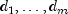,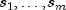(m is number of differences or `sIinitial.length`) and p in the AR model: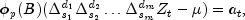where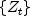is the original time series, B is the backward shift operator defined by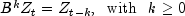,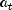is Gaussian white noise with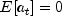and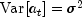,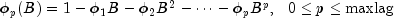,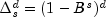, with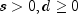, and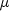is a centering parameter for the differenced series.

Returns:
a `double` array of length `z.length` or `z.length-nLost`, depending upon the setting for `setExclude`
Throws:
`ARMA.MatrixSingularException` - is thrown if the input matrix is singular
`ARMA.TooManyCallsException` - is thrown if the number of calls to the function has exceeded the maximum number of iterations times the number of moving average (MA) parameters + 1.
`ARMA.IncreaseErrRelException` - is thrown if the bound for the relative error is too small
`ARMA.NewInitialGuessException` - is thrown if the iteration has not made good progress
`ARMA.IllConditionedException` - is thrown if the problem is ill-conditioned
`ARMA.TooManyITNException` - is thrown if the maximum number of iterations is exceeded
`ARMA.TooManyFcnEvalException` - is thrown if the maximum number of function evaluations is exceeded
`ARMA.TooManyJacobianEvalException` - is thrown if the maximum number of Jacobian evaluations is exceeded
`ARMAMaxLikelihood.NonStationaryException` - is thrown if the final maximum likelihood estimates for the time series are nonstationary
`ARMAMaxLikelihood.NonInvertibleException` - is thrown if the final maximum likelihood estimates for the time series are noninvertible
`ARMAMaxLikelihood.InitialMAException` - is thrown if the initial values provided for the moving average terms using ``` setMA``` are noninvertible. In this case, ``` ARMAMaxLikelihood ``` terminates and does not compute the time series estimates.
`ARAutoUnivariate.TriangularMatrixSingularException` - is thrown if the input triangular matrix is singular.
• #### setCenter

`public void setCenter(int center)`
Controls centering of the differenced series.
Parameters:
`center` - an `int` scalar value equal `NO_CENTER` , ``` CENTER_MEAN```, or ```CENTER_MEDIAN ```. By default, `center=CENTER_MEAN`
• #### setDInitial

`public void setDInitial(int[][] dInitial)`
Sets the candidate values for selecting the optimum seasonal adjustment prior to calling the compute method.
Parameters:
`dInitial` - an `int` array of candidate values for d to evaluate. All values must be non-negative. `dInitial` must have the same number of differences (columns) as `sInitial`. By default, `dInitial` is initialized to all ones.
• #### setExclude

`public void setExclude(boolean exclude)`
Controls whether to exclude or replace the inital values in the transformed series.

If exclude is `true`, then inital values in the transformed series that cannot be computed are set to missing, `NaN`. This ensures that the length of the transformed seriesis equal to the length of the time series, ```z.length ```. If exclude is set to `false`, then inital values in the transformed seriesthat cannot be computed are removed. This makes the length of the transformed seriesequal to `z.length-`nLost where nLost is the number of lost values obtained from method `getNLost`.

Parameters:
`exclude` - a `boolean` value that controls whether values inthat cannot be calculated are dropped or set to missing. Missing values are set to `Double.NaN`. By default, `exclude` =`true`
JMSLTM Numerical Library 7.2.0